# Weil's positivity criterion

André Weil [ MR 14,727e] proved the following explicit formula (see also A. P. Guinand [ MR 10,104g] which specifically illustrates the dependence between primes and zeros. Let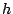be an even function which is holomorphic in the strip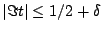and satisfying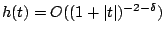for some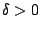, and let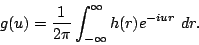Then we have the following duality between primes and zeros: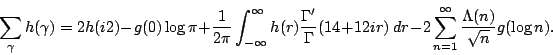In this formula, a zero is written as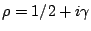where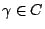; of course RH is the assertion that all of the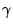are real. Using this duality Weil gave a criterion for RH.

Back to the main index for The Riemann Hypothesis.